+关注继续查看

# [C++ 面试基础知识总结]字符串，向量和数组

## string

### string的读写

#include <iostream>
using namespace std;

int main(int argc, const char * argv[]) {
string s;
cin >> s;
cout << s << endl;
return 0;
}

### string::size_type类型

string类型的size()函数返回类型为string::size_type，这个类型是一个无符号类型的值，且能足够放下任何string对象的大小。所以尽量不要在一个表达式中混用string::size_type和int，不然可能会带来问题。

#include <iostream>
using namespace std;

int main(int argc, const char * argv[]) {
string s = "Hello";
int n = -1;
bool flag = s.size() < n;
cout << flag << endl;
return 0;
}

### string对象和字面值相加

string s1 = "Hello", s2 = "World";
//正确
string s3 = s1 + "!" + s2;
//错误 不能把字符串字面值直接相加
string s4 = "Hello" + "!" + s2;
//正确
string s5 = "Hello" + ("!" + s2);

## vector容器

### vector的初始化

// v1有10个元素，每个元素的值都为0（int类型的默认初始值）
vector<int> v1(10);
// v2有1元素，值为10
vector<int> v2{10};
// v3有10个元素，每个元素值都为1
vector<int> v3(10,1);
// v4有2个元素，值分别为10和1
vector<int> v4{10,1};

// 错误，不能用字面值来构建vector对象
vector<string> v1("hi");
// v2有1元素，值为"hi"，列表初始化
vector<string> v2{"hi"};
// v3有10个元素，每个元素值都为空string对象，不能列表初始化，用默认值构造vector对象
vector<string> v3{10};
// v3有10个元素，每个元素值都为"hi"，不能列表初始化，用提供的值构造vector对象
vector<string> v4{10,"hi"};

### 使用vector的注意事项

vector的size()函数与string的同名函数功能完全一致，但要使用size_type时，需指定它是由哪种类型定义的，vector对象的类型总是包含着元素的类型。

vector<int>::size_type //正确
vector::size_type      //错误

vector<int> v;
for (decltype(v.size()) i = 0; i != 10; i++) {
v[i] = 1; //错误，v初始为空，不包含任何元素
}
vector<int> v;
for (decltype(v.size()) i = 0; i != 10; i++) {
v.push_back(1); //正确
}

## 迭代器

### 迭代器运算符

#include <iostream>
using namespace std;

int main(int argc, const char * argv[]) {
string s("Hello");
//确保字符串s非空
if (s.begin() != s.end()) {
auto t = s.begin();
++*t;
cout << *t << endl;
++t;
if (t != s.end()) {
cout << *t << endl;
}
}
return 0;
}

++*t是将t指向的字符加1，而++t是将t移动到下一个元素。所以两次输出的结果分别为I（第一个字符H加1），e（第二个字符）。

### 使用迭代器实现二分查找

#include <iostream>
#include <vector>
using namespace std;

int main(int argc, const char * argv[]) {
vector<int> v{1,2,3,4,5,6,7,8,9,10};
auto begin = v.begin(), end = v.end();
auto mid = begin + (end - begin)/2;
while (mid != end) {
cout << *mid << endl;
if (*mid == 2) {
cout << "success" << endl;
break;
}
else if (*mid > 2){
end = mid;
}
else{
begin = mid + 1;
}
mid = begin + (end - begin)/2;
}
return 0;
}

6
3
2
success

## 数组

### 初始化和赋值

unsigned sz1 = 10;
constexpr unsigned sz2 = 10;
// 正确，声明一个含有10个整数的数组
int a1;
// 错误，sz1不是常量表达式
int a2[sz1];
// 正确，声明一个含有10个整数的数组
int a3[sz2];
// 错误，未初始化维度
int a4[];
// 正确，维度是3的数组
int a5[] = {0,1,2};
// 错误，不能用数组内容拷贝给其他数组作为其初始值，也不能用数组为其他数组赋值
int a6[] = a5;

### 字符数组

// 列表初始化，没有空字符
char a1[] = ['C','+','+'];
// 列表初始化，含有显式空字符
char a2[] = ['C','+','+','\0'];
// 自动添加结尾处的空字符
char a3[] = "C++";
// 错误，没有空间存放空字符
char a4 = "C++";

### 数组与指针

// 含有10个整型指针的数组
int *p1;
// 指向一个含有10个整数的数组的指针
int (*p2);
// 指向一个含有10个整型指针的数组的指针
int *(*p3);

int a = {1,2};
//因为无法用数组的内容初始化另一个数组，所以用auto可以推断出p4类型为整型指针，指向a的第一个元素
auto p4 = a;

int a = {0,2,4,6,8};

// 以下3种写法等价，都是将指针指向数组的第一个元素
int *p1 = a;
int *p2 = &a;
int *p3 = begin(a);

// n1值为8（将p1前进4个元素后解引的值即a）
int n1 = *(p1 + 4);
// n2值为4（将p1解引后加4的值即a+4）
int n2 = *p1 + 4;

### C风格字符串

char a[] = {"C","+","+"};
// strlen函数是计算字符串长度的，这里会出错，因为没有以空字符结尾，函数将可能沿着数组的内存位置不断向前寻找，直到遇到空字符才停下来。
count << strlen(a) << endl;

char s1[] = "Hello", s2[] = "World";
//错误，这里比较的将是s1和s2的地址，而不是字符串本身
if (s1 < s2)
//正确比较字符串
if (strcmp(s1,s2))

//错误，s1和s2是两个指针相加
char s3[] = s1 + s2;
//声明一个足够放下s1和s2的字符数组，如果无法估计准确，会出现安全风险
char s4;
//将s1复制到s4之后返回s4
strcpy(s4,s1);
//将s2加到s4之后返回s4
strcat(s4,s2);

### 多维数组中的指针

#include <iostream>

int main(int argc, const char * argv[]) {

int a = {{0,2,4},{6,8,10}};
// 等价于 int (*p) = a,p指向含有3个整数的数组
auto p = a;
cout << **(p+1) << endl;
cout << *(*p+1) << endl;
cout << (**p+1) << endl;
return 0;
}

6
2
1

**(p+1)是将指针p向后推进1个元素再进行两次解引，第一解引结果*(p+1)为指向数组{6，8，10}首元素的指针，再次解引则为整数6。
*(*p+1)是先将p进行解引，解引结果*p为指向数组{0,2,4}首元素的指针，往后推进1个元素后指向第二个元素，解引得整数2。
(**p+1)是将p连续解引两次，得到数组{0,2,4}首元素0，加1后得到整数1。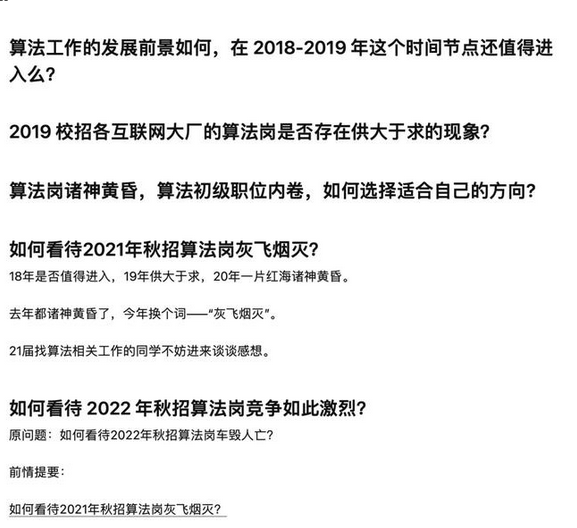27 0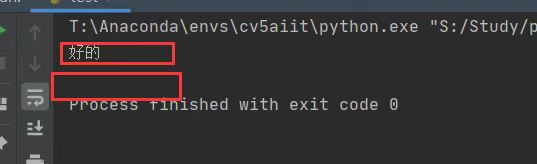python中字符串数据处理的那些方法------=为了面试武装到牙齿=----==《《满满的硬货》》-----====实用派（2）
python中字符串数据处理的那些方法------=为了面试武装到牙齿=----==《《满满的硬货》》-----====实用派（2）
52 0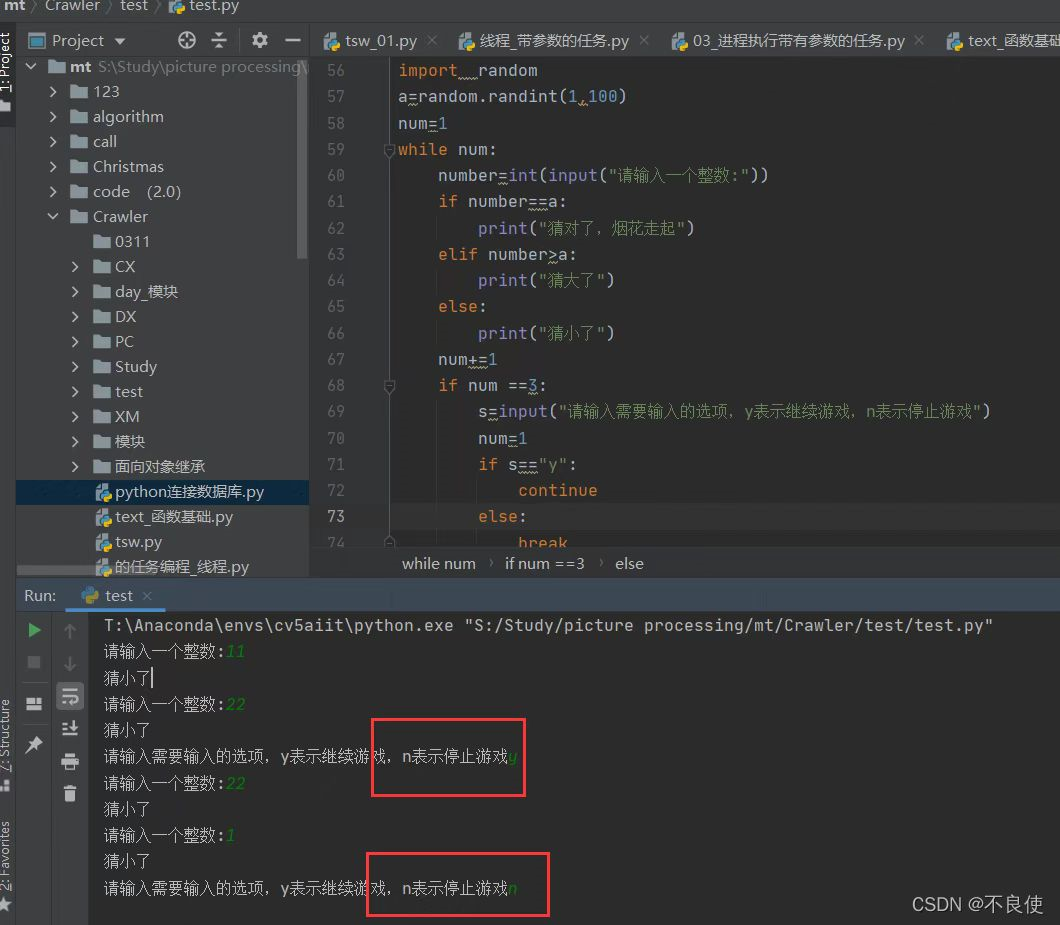python中字符串数据处理的那些方法------=为了面试武装到牙齿=----==《《满满的硬货》》-----====实用派（1）
python中字符串数据处理的那些方法------=为了面试武装到牙齿=----==《《满满的硬货》》-----====实用派（1）
51 0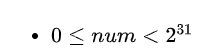【每日算法Day 86】面试经典题：把数字翻译成字符串

48 0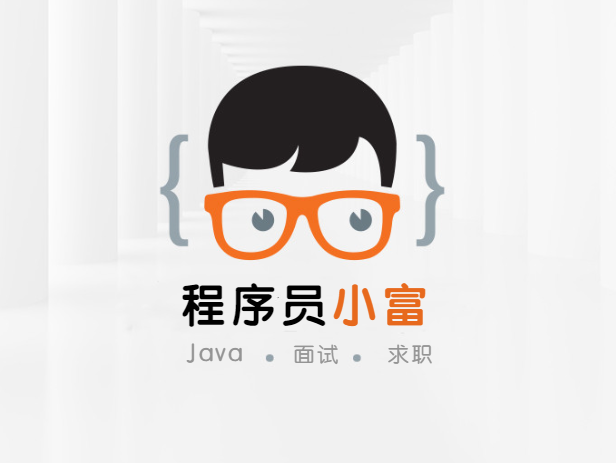64 0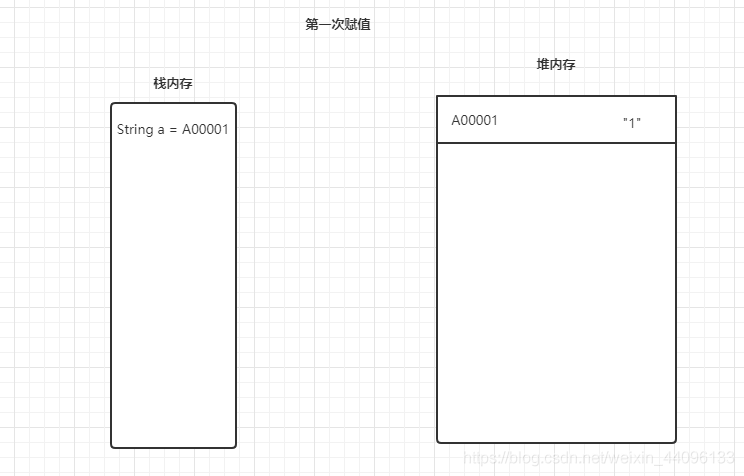java 中操作字符串都有哪些类？它们之间有什么区别？面试篇（第七天）
String：对字符串不进行重复操作时选择用String。 StringBuilder：在单线程中对字符串进行重复操作时选择用StringBuilder。 StringBuffer：在多线程中对字符串进行重复操作时选择用StringBuffer。
65 048 0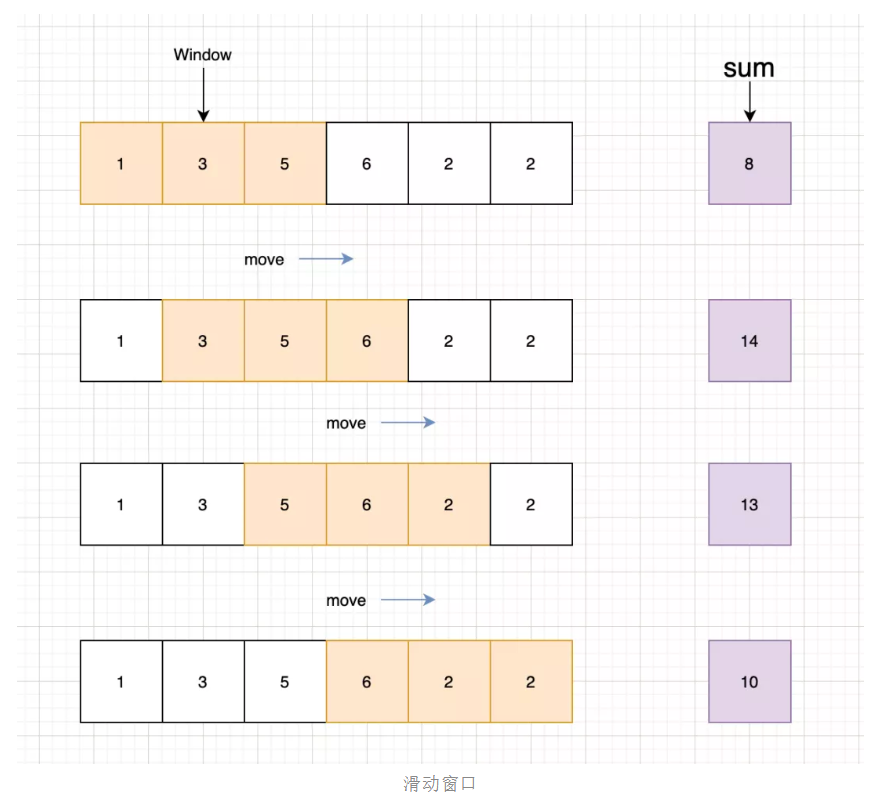40 0

Go语言核心编程 - 基础语法、数组、切片、Map2806182

Java开发者面试百宝书